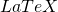# Adding LATEX to posts

WordPress supports, a typesetting system that’s really good at formatting mathematical formulas and equations.

#### Using LaTeX

To includecode in your post, use the following:

$latex your-latex-code-here$

So, for example,

$latex ihbarfrac{partial}{partial t}left|Psi(t)right>=Hleft|Psi(t)right>$

produces

$latex i\hbar\frac{\partial}{\partial t}\left|\Psi(t)\right>=H\left|\Psi(t)\right>$

#### LaTeX Error

If yourcode is broken, instead of the equation you’ll see an ugly yellow and red error message. One thing to keep in mind is that WordPress puts all of yourcode inside amath environment. If you try to usethat doesn’t work inside the math environment (such as begin{align} ... end{align}), you will get an error:$LaTeX&s=X$

#### LaTeX Size

You can change the size of theby specifying an s parameter after thecode.

$latex LaTeX&s=X$

Where X goes from -4 to 4 (0 is the default). These sizes correspond to‘s font size commands:

S Font Size Example
-4 tiny $latex LaTeX&s=-4$
-3 scriptsize $latex LaTeX&s=-3$
-2 footnotesize $latex LaTeX&s=-2$
-1 small $latex LaTeX&s=-1$
0 normalsize (12pt) $latex LaTeX&s=0$
1 large $latex LaTeX&s=1$
2 Large $latex LaTeX&s=2$
3 LARGE $latex LaTeX&s=3$
4 huge $latex LaTeX&s=4$

#### LaTeX Colors

WordPress tries to guess the background and foreground colors of your site and generates theimage accordingly. But, you can change the colors. You can specify bg and fg parameters after thecode to change the background and foreground colors, respectively. The colors must be in hexadecimal RGB format: ffffff for white, 0000ff for bright blue, etc.

$latex LaTeX&bg=ffcccc&fg=cc00ff&s=4$

$latex LaTeX&bg=ffcccc&fg=cc00ff&s=4$

#### LaTeX Packages

WordPress uses standardwith the following packages:

• amsmath
• amsfonts
• amssymb

#### More Information

For more information about, you can visit LaTeX documentation site and TeX Resources by American Mathematical Society.Nanomembrane Research Group Webadmin.